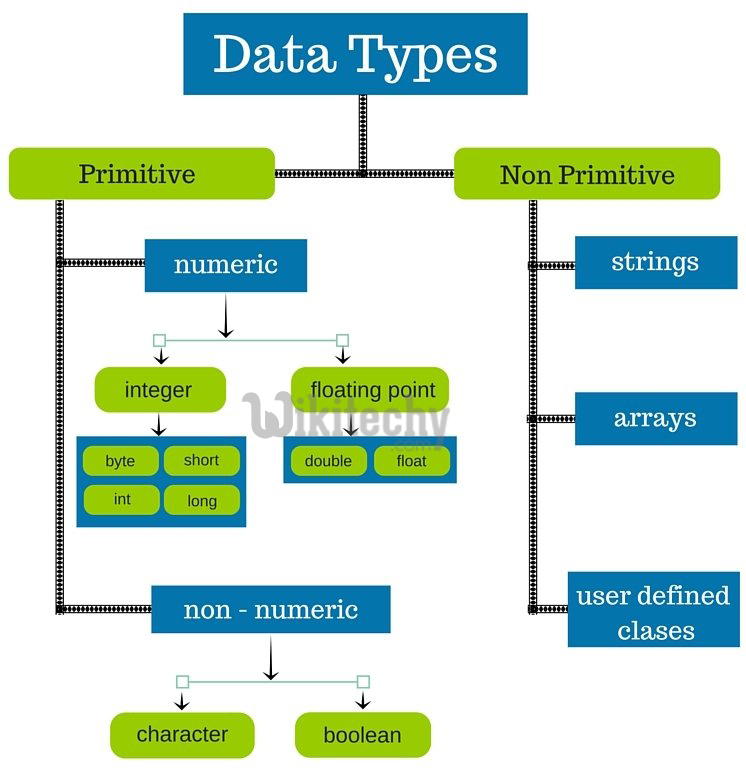# golang tutorial - Data types - golang - go programming language - google go - go language - go program - google language

## Below are the data types in Go language

S.N. Types and Description
1. Boolean Types
They are boolean types and consists of the two predefined constants:
(a) true
(b) false
2. Numeric Types
They are again arithmetic types and they represents
a) integer types or
b) floating point values.
3. String Types:
• A string type represents the set of string values. Its value is a sequence of bytes.
• Strings are immutable types that is once created, it is not possible to change the contents of a string. The predeclared string type is string.
4. Derived Types:
(a) Pointer types,
(b) Array types,
(c) Structure types,
(d) Union types and
(e) Function types
f) Slice types
g) Function types
h) Interface types
i) Map types
j) Channel Types## Integer Types

• Below is the sub classification in integer types
S.N. Types and Description
1. uint8
Unsigned 8-bit integers (0 to 255)
2. uint16
Unsigned 16-bit integers (0 to 65535)
3. uint32
Unsigned 32-bit integers (0 to 4294967295)
4. uint64
Unsigned 64-bit integers (0 to 18446744073709551615)
5. int8
Signed 8-bit integers (-128 to 127)
6. int16
Signed 16-bit integers (-32768 to 32767)
7. int32
Signed 32-bit integers (-2147483648 to 2147483647)
8. int64
Signed 64-bit integers (-9223372036854775808 to 9223372036854775807)

## Floating Types

• Below is the sub classification in float types
S.N. Types and Description
1. float32
IEEE-754 32-bit floating-point numbers
2. float64
IEEE-754 64-bit floating-point numbers
3. complex64
Complex numbers with float32 real and imaginary parts
4. complex128
Complex numbers with float64 real and imaginary parts
• The value of an n-bit integer is n bits and is represented using two's complement arithmetic operations.

## Other Numeric Types

• Below is the sub classification in numeric types,
S.N. Types and Description
1. byte
same as uint8
2. rune
same as int32
3. uint
32 or 64 bits
4. int
same size as uint
5. uintptr
an unsigned integer to store the uninterpreted bits of a pointer value